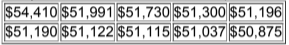×
Get Full Access to Elementary Statistics - 12 Edition - Chapter 3.2 - Problem 6bsc
Get Full Access to Elementary Statistics - 12 Edition - Chapter 3.2 - Problem 6bsc

×

# Solved: In Exercises, find the (a)mean, (b) median, (c)ISBN: 9780321836960 18

## Solution for problem 6BSC Chapter 3.2

Elementary Statistics | 12th Edition

• Textbook Solutions
• 2901 Step-by-step solutions solved by professors and subject experts
• Get 24/7 help from StudySoup virtual teaching assistantsElementary Statistics | 12th Edition

4 5 1 291 Reviews
11
0
Problem 6BSC

Top 10 Most Expensive Colleges Listed below are the annual tuition amounts of the 10 most expensive colleges in the United States for a recent year. The colleges listed in order are Sarah Lawrence, NYU, George Washington, Bates, Skidmore, Johns Hopkins, Georgetown, Connecticut College, Harvey Mudd, and Vassar. What does this “Top 10” list tell us about the population of all U.S. college tuitions?Step-by-Step Solution:

Step 1 of 1

(a) The "Mean" is computed by adding all of the numbers in the data together and dividing by the number elements contained in the data set.

Mean == $51596.6 (b) The "Median" of a data set is dependant on whether the number of elements in the data set is odd or even. First reorder the data set from the smallest to the largest then if the number of elements are odd, then the Median is the element in the middle of the data set. If the number of elements are even, then the Median is the average of the two middle terms.  50875 51037 51115 51122 51190 51196 51300 51730 51991 54410 Median ==$51193

(c) The "Mode" for a data set is the element that occurs the most often. It is not uncommon for a data set to have more than one mode. This happens when two or more elements occur with equal frequency in the data set. A data set with two modes is called bimodal. A data set with three modes is called trimodal.

Since there is no repeated terms in the data. Hence there is no mode.

(d) In statistics, the mid-range or mid-extreme of a set of statistical data values is the arithmetic mean of the maximum and minimum values in a data set, defined as

Mid range =\$52642.5

Step 2 of 1

##### ISBN: 9780321836960

Unlock Textbook Solution